# R S Aggarwal Solutions for Class 11 Maths Chapter 24 Hyperbola

R S Aggarwal Solutions Class 11 Maths help students to learn various Maths concepts covered under the textbook. Chapter 24 of RS Aggarwal Solutions for Class 11 Maths is based on Hyperbola and terms related to it such as centre, axes, vertices, length of axes, eccentricity, length of latus rectum and many more. R S Aggarwal Class 11 Solutions are prepared by BYJU’S subject experts to help students clear their concepts and score good marks in exams.

Important points to remember:

1. Horizontal Hyperbola:

For general form of Hyperbola:

x2/a2 – y2/b2 = 1

 Length of Transverse axis Length of Conjugate axis vertices foci eccentricity latus rectum 2a 2b (±a, 0) (±c, 0) e = c/a 2b2/a

2. Vertical Hyperbola:

For general form of Hyperbola:

y2/a2 – x2/b2 = 1

 Length of Transverse axis Length of Conjugate axis vertices foci eccentricity latus rectum 2a 2b (0, ±a) (0, ±c) e = c/a 2b2/a

## Download PDF of R S Aggarwal Solutions for Class 11 Maths Chapter 24 Hyperbola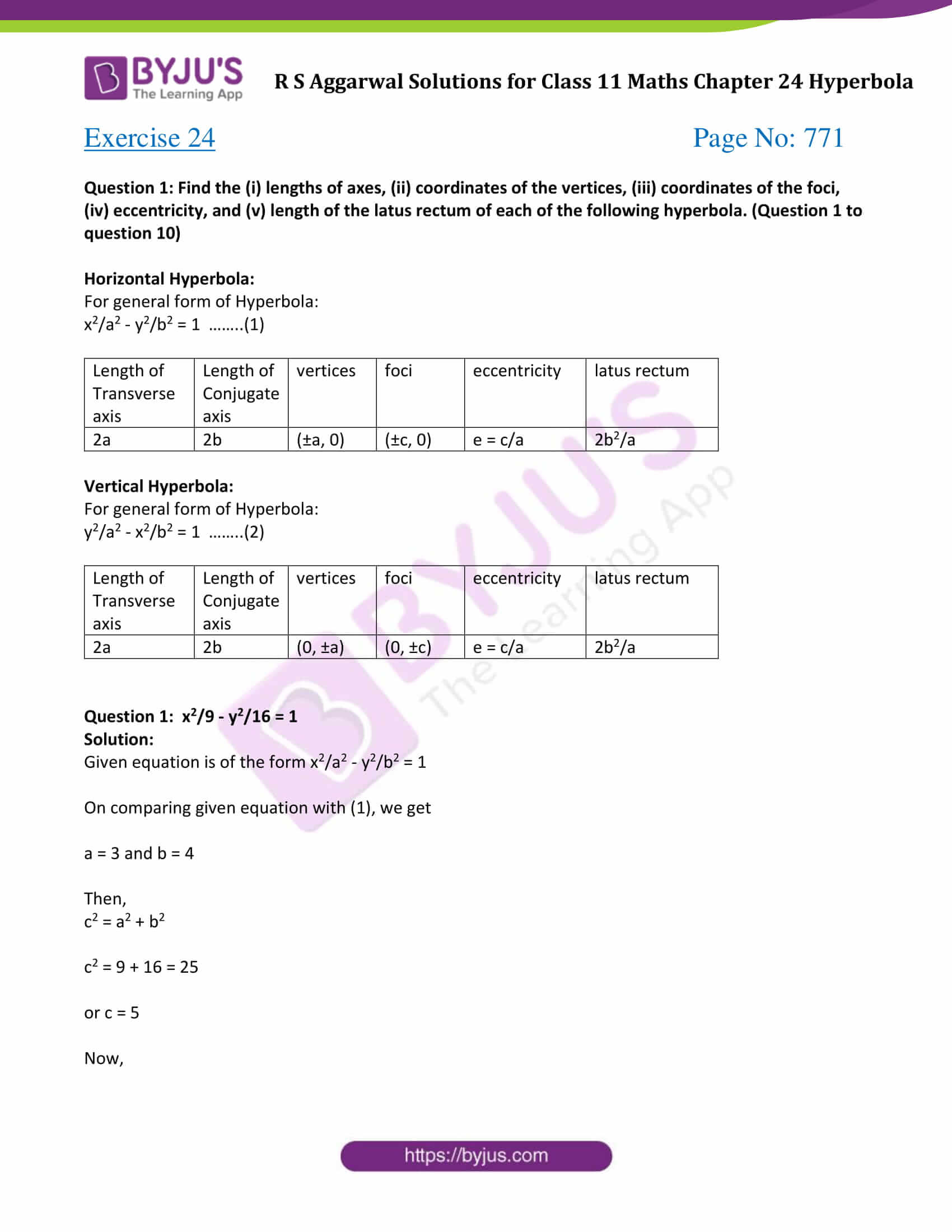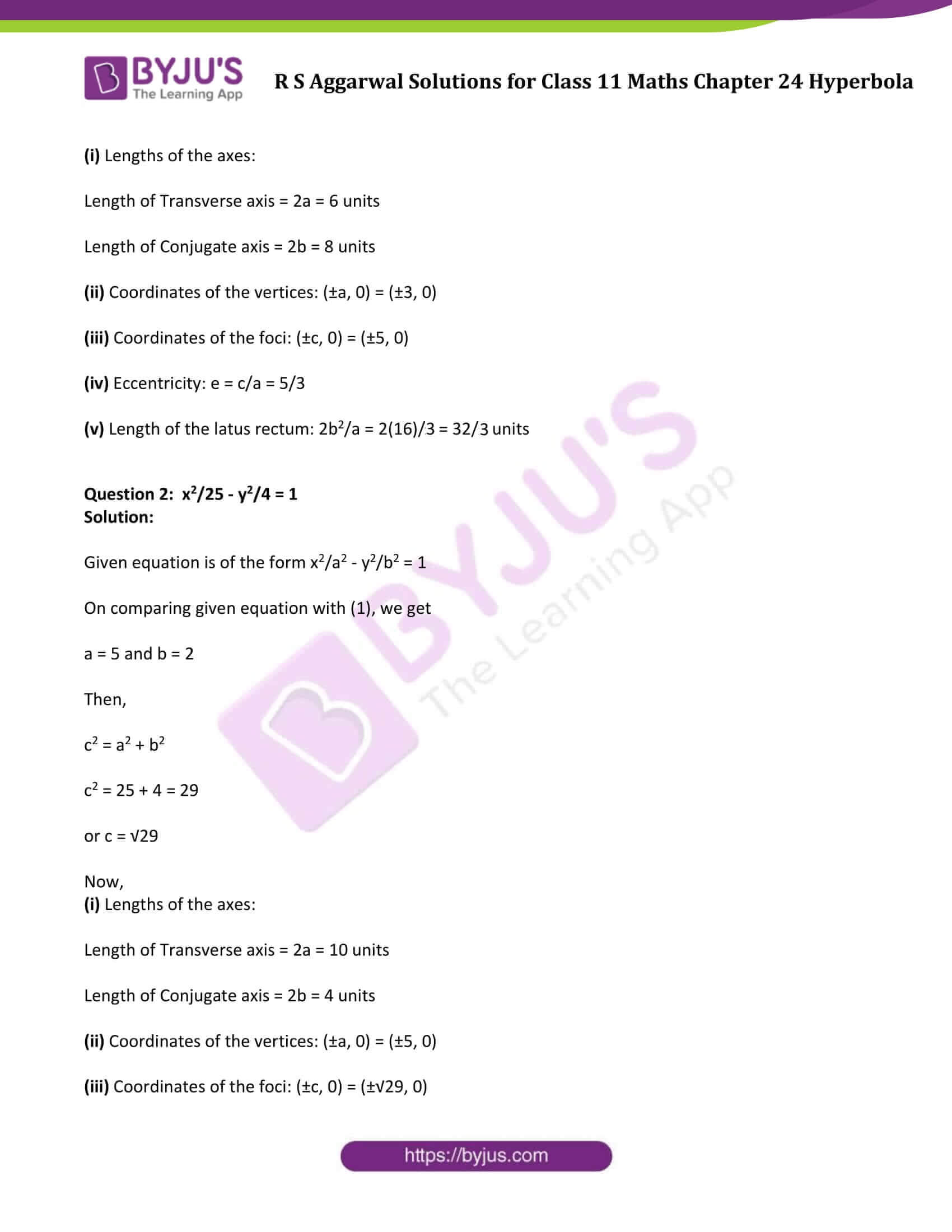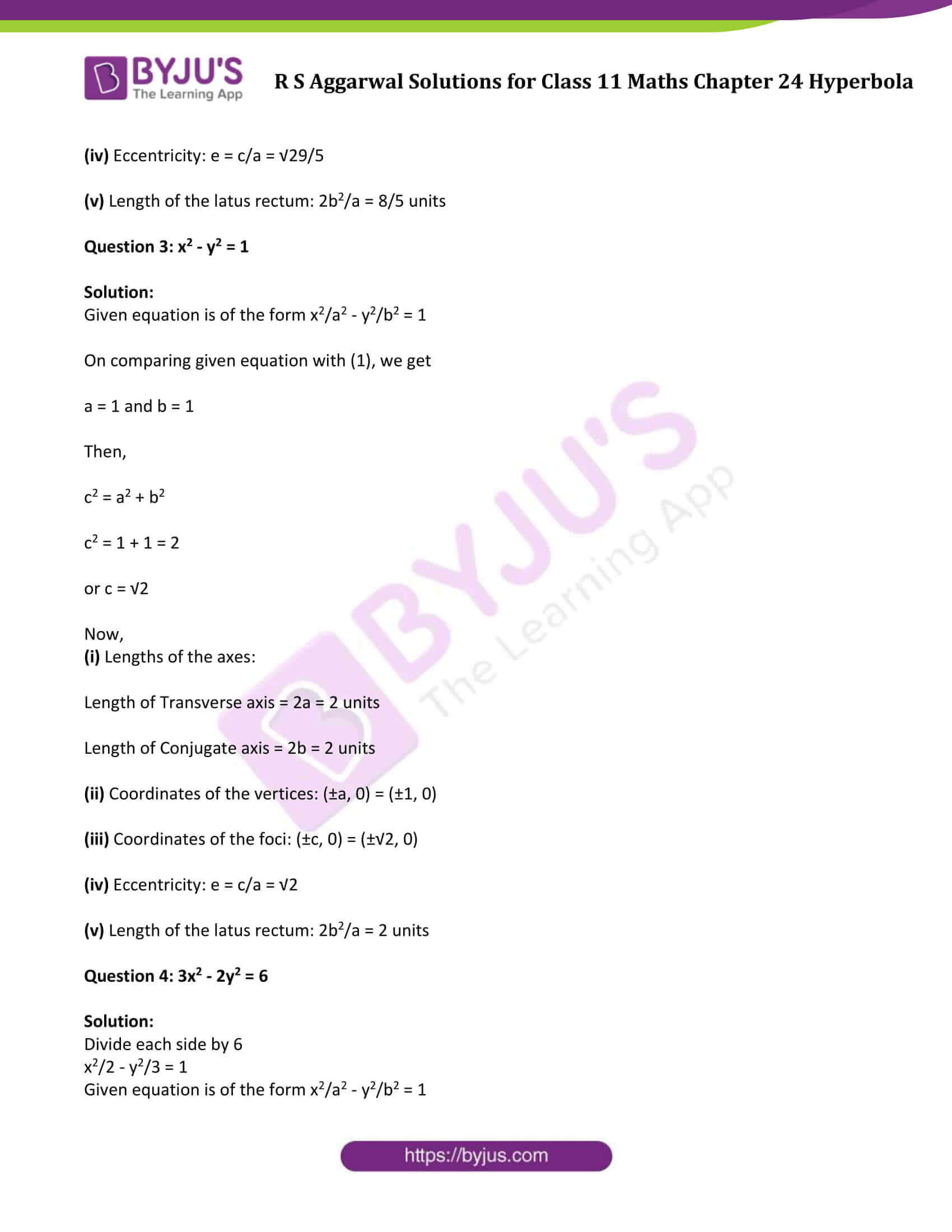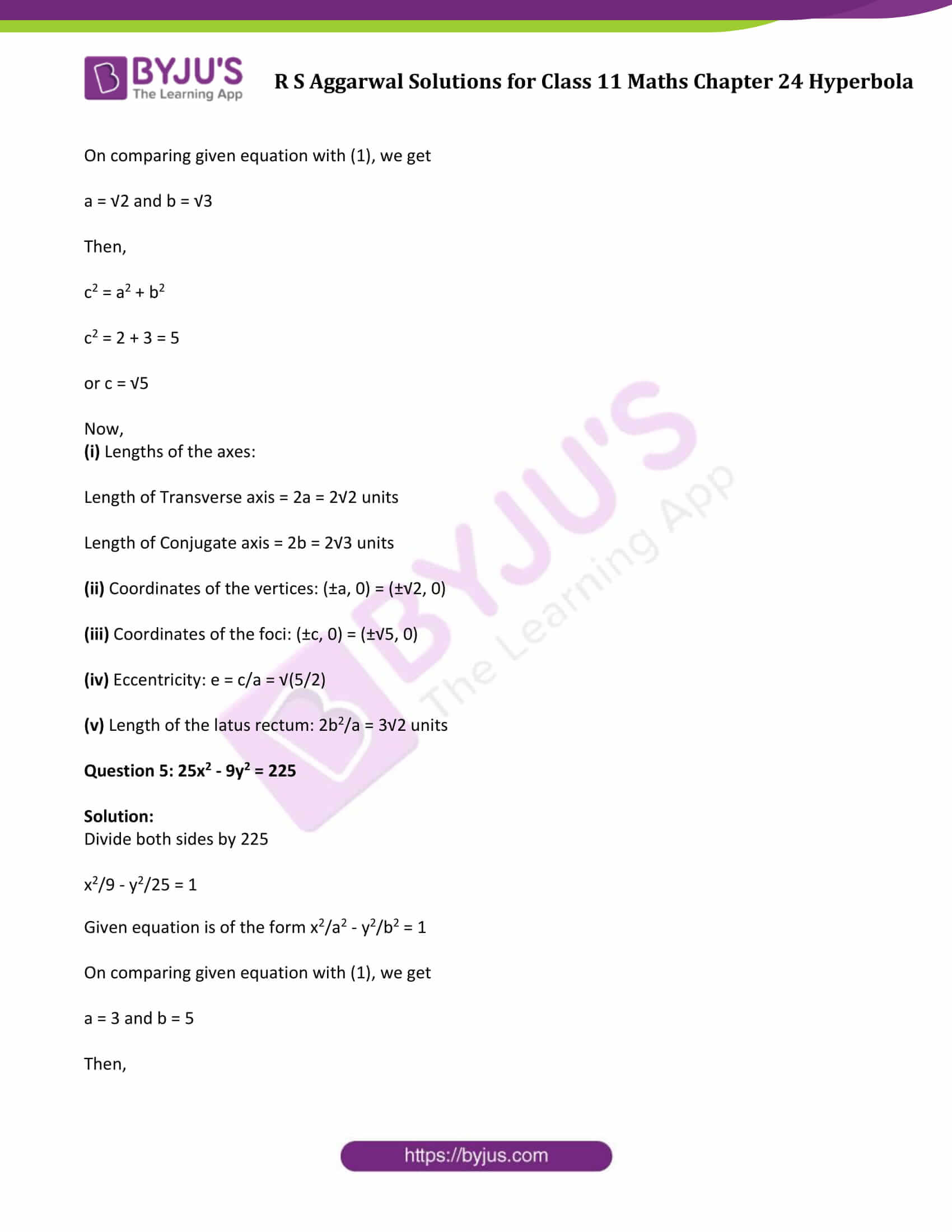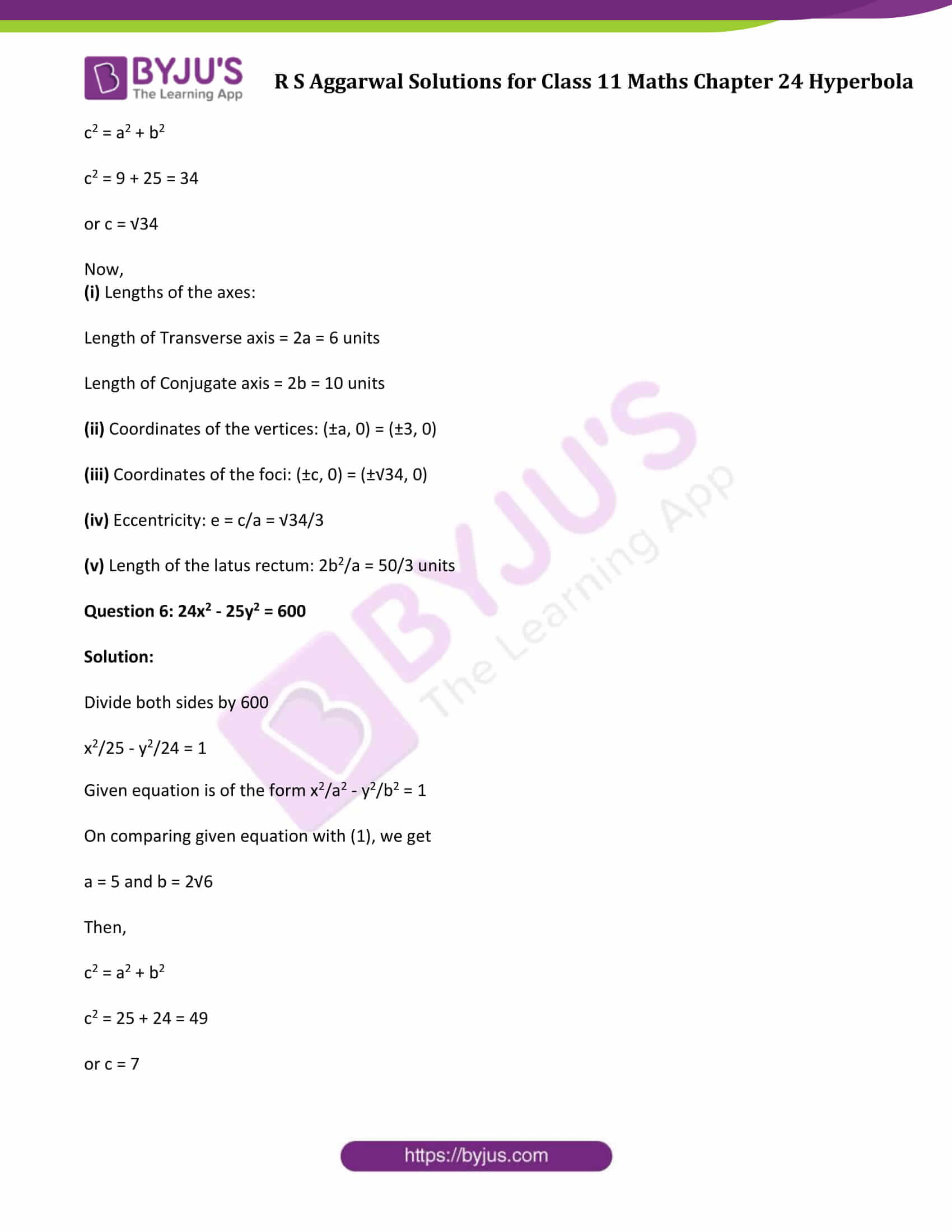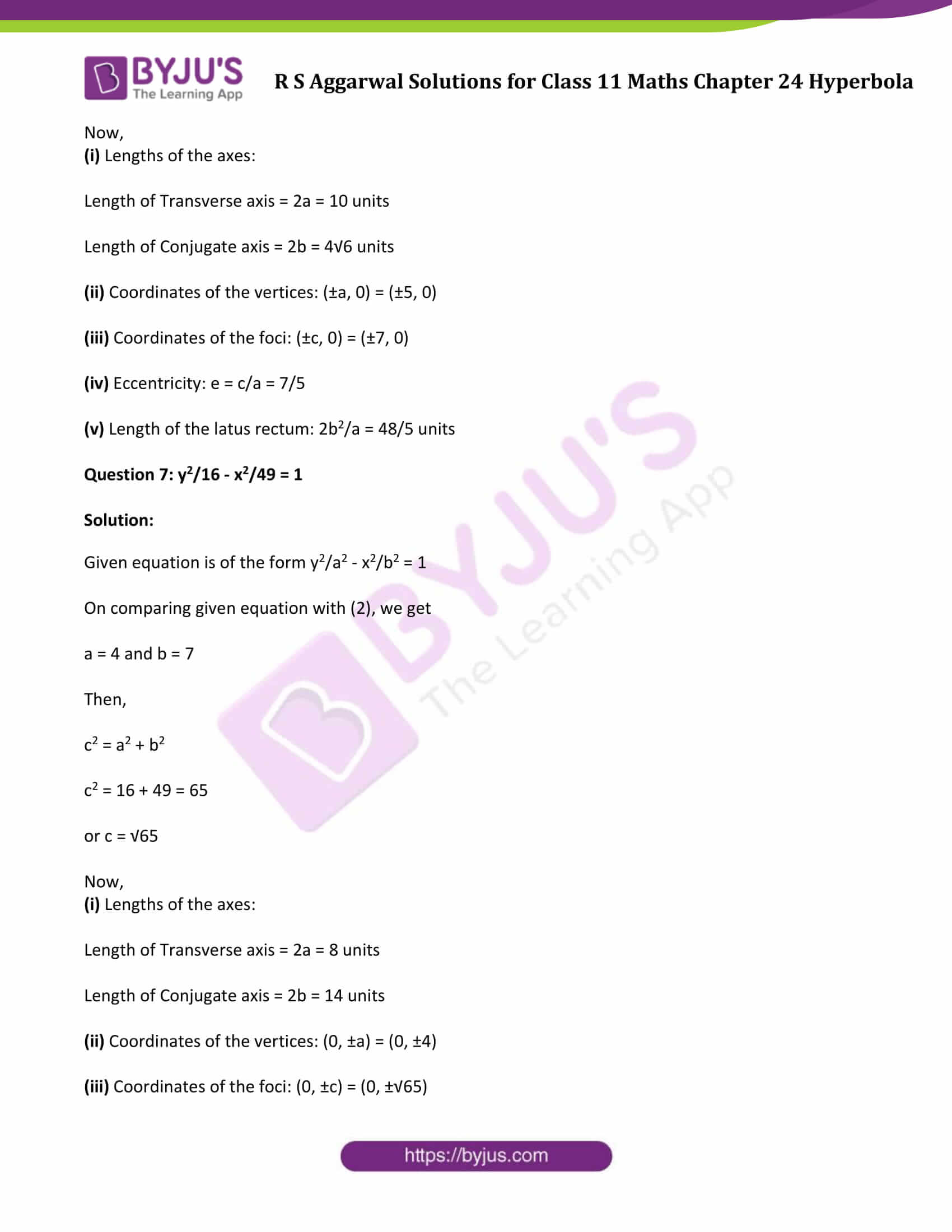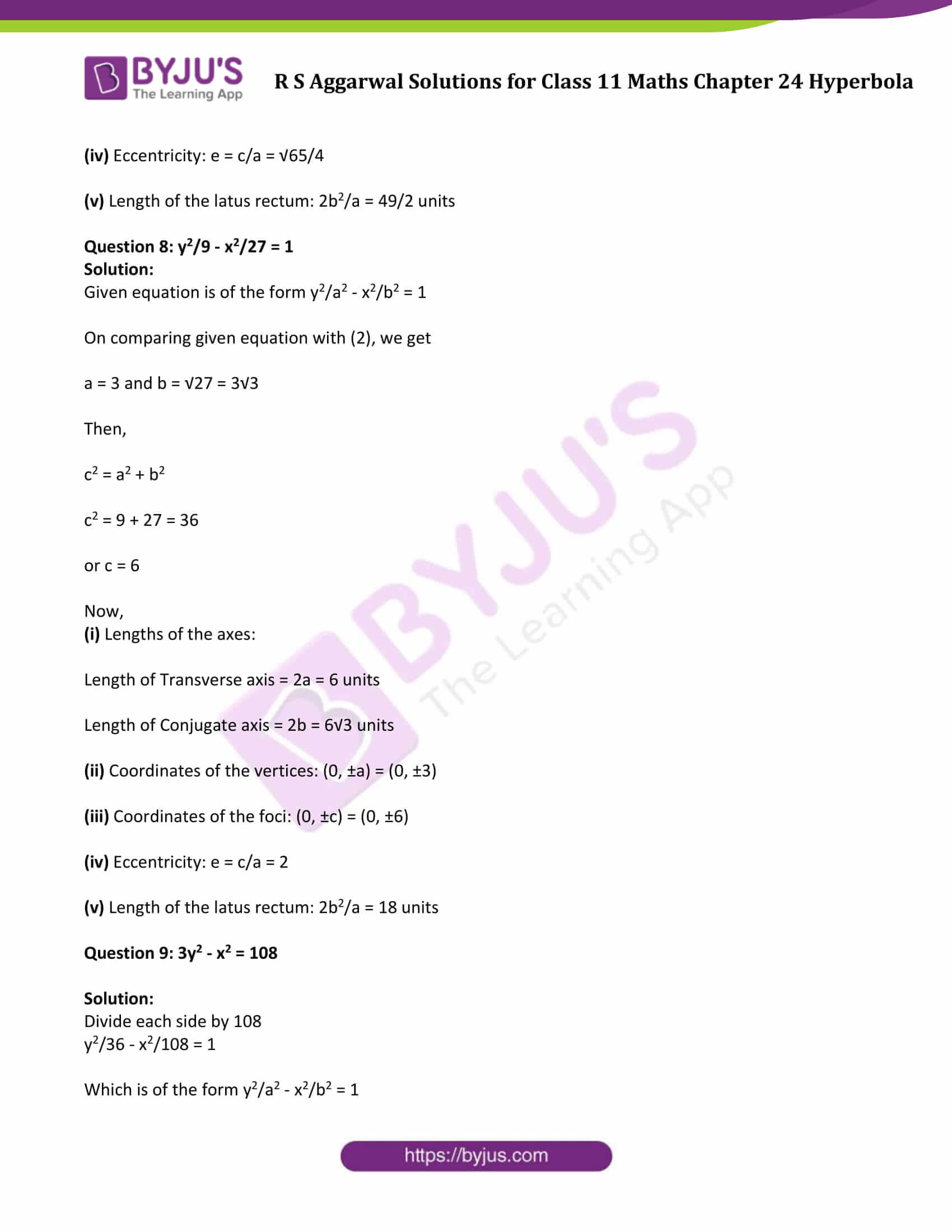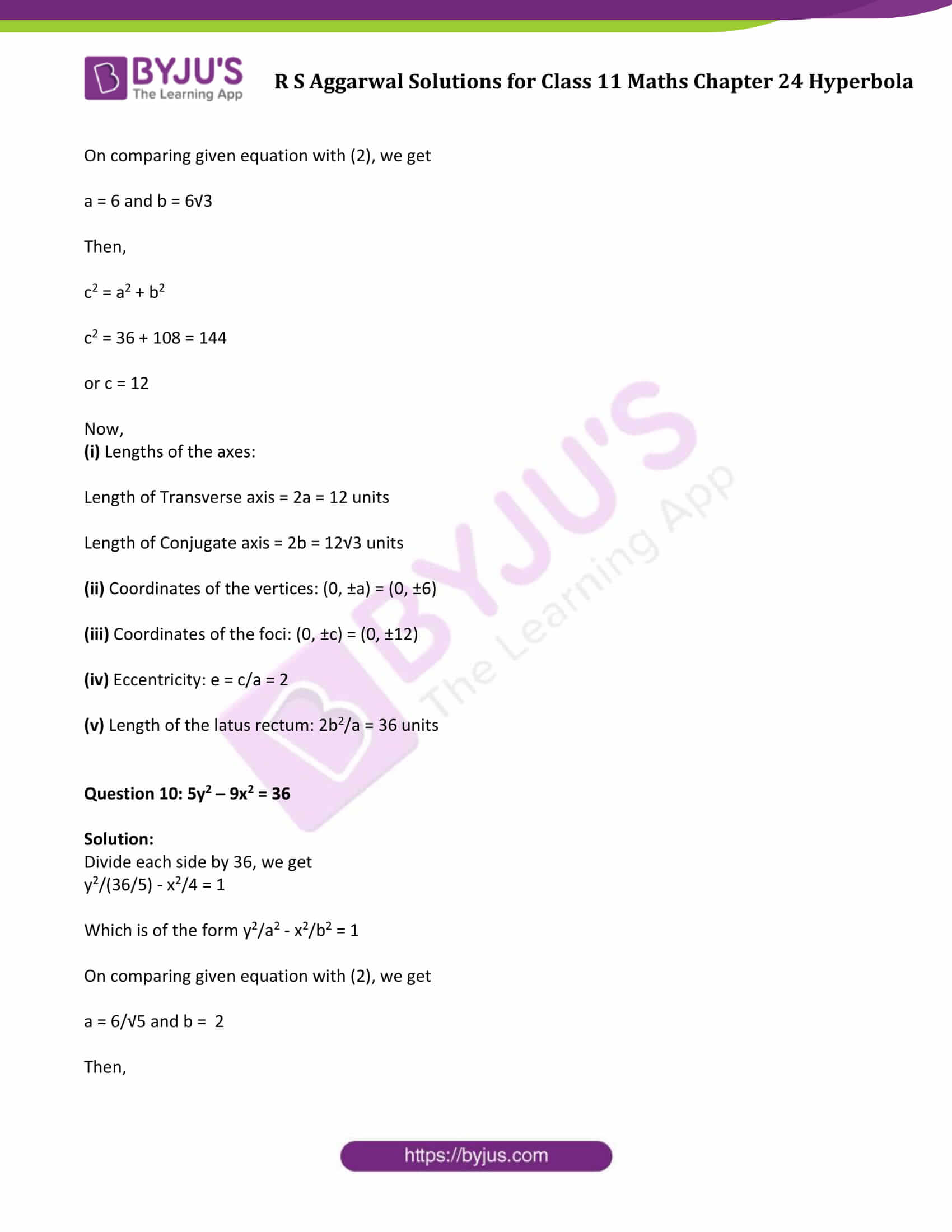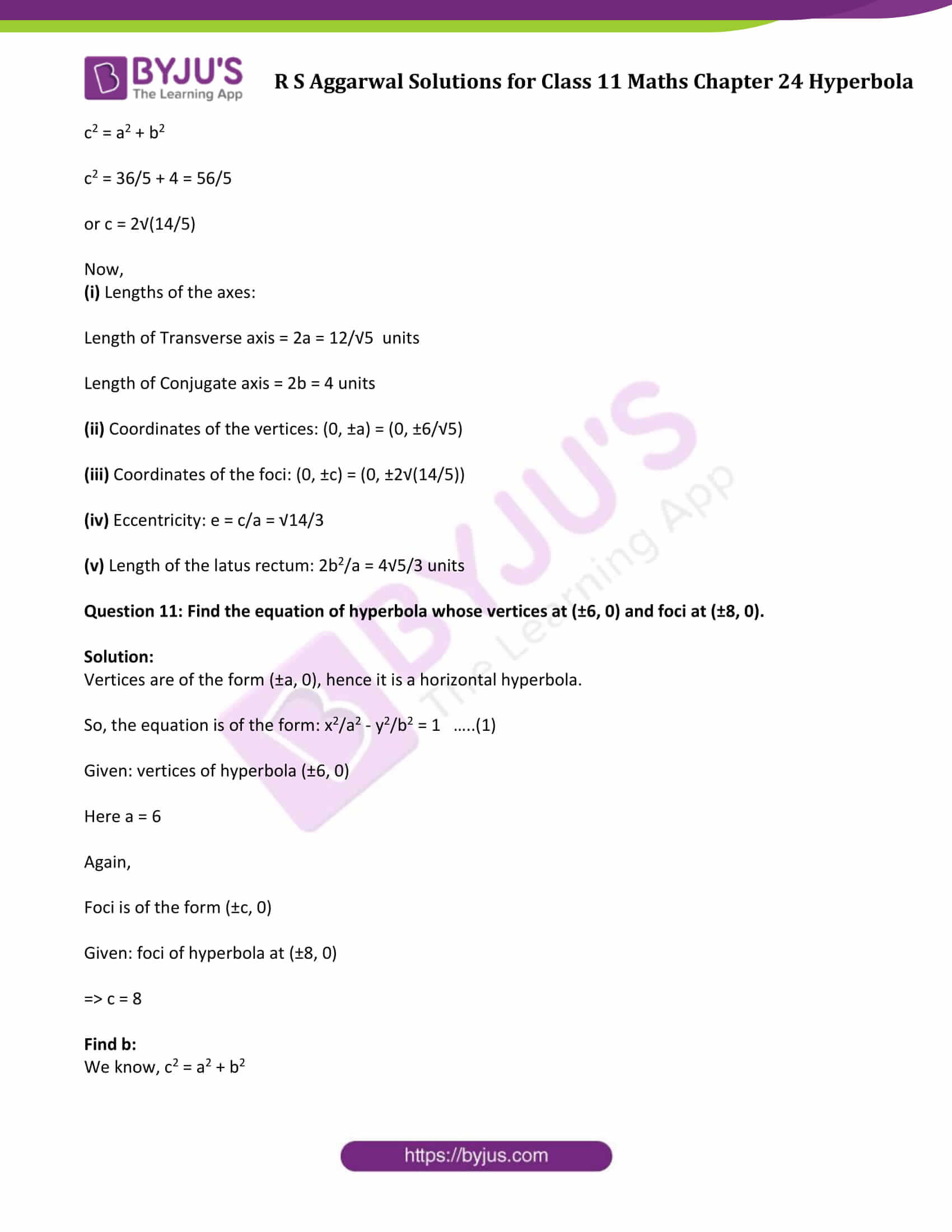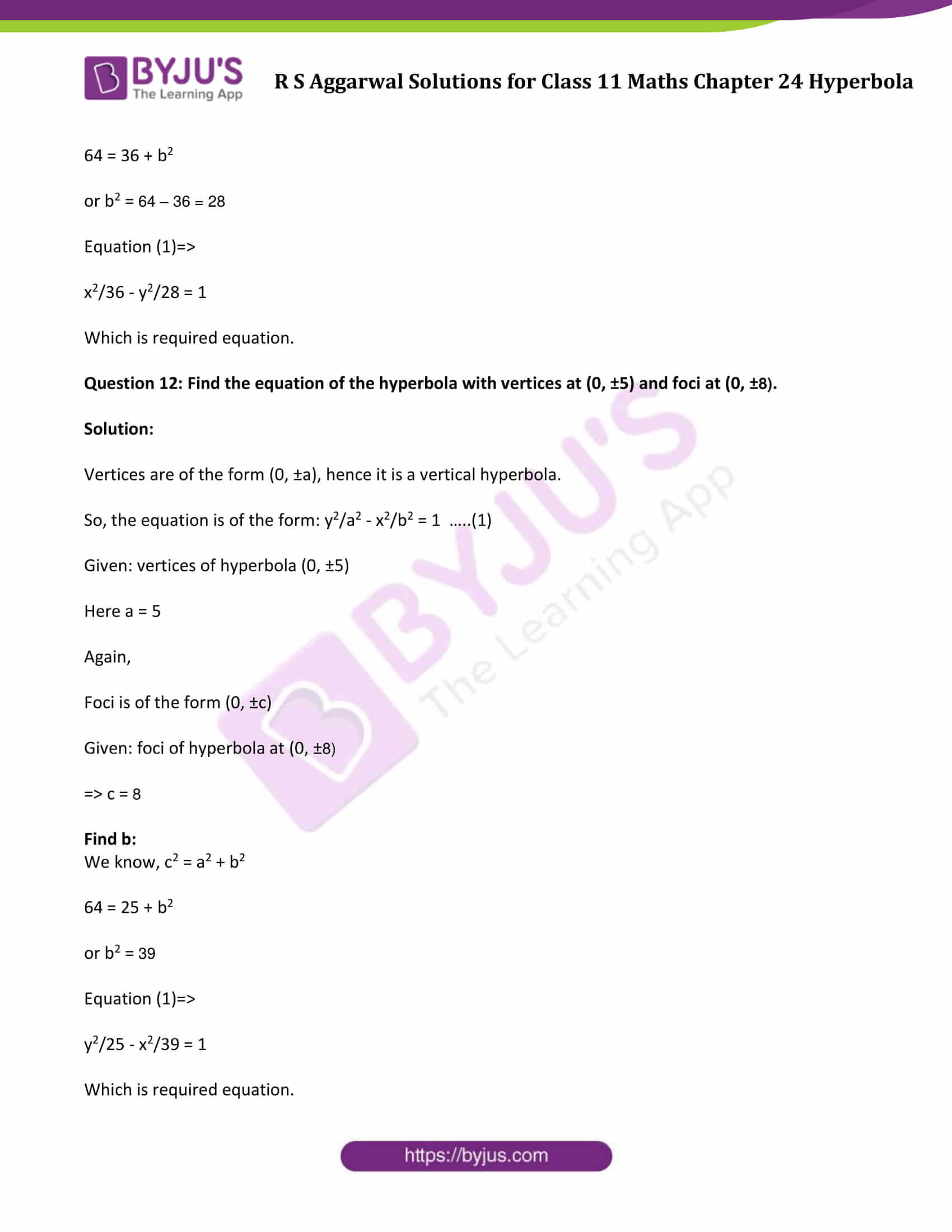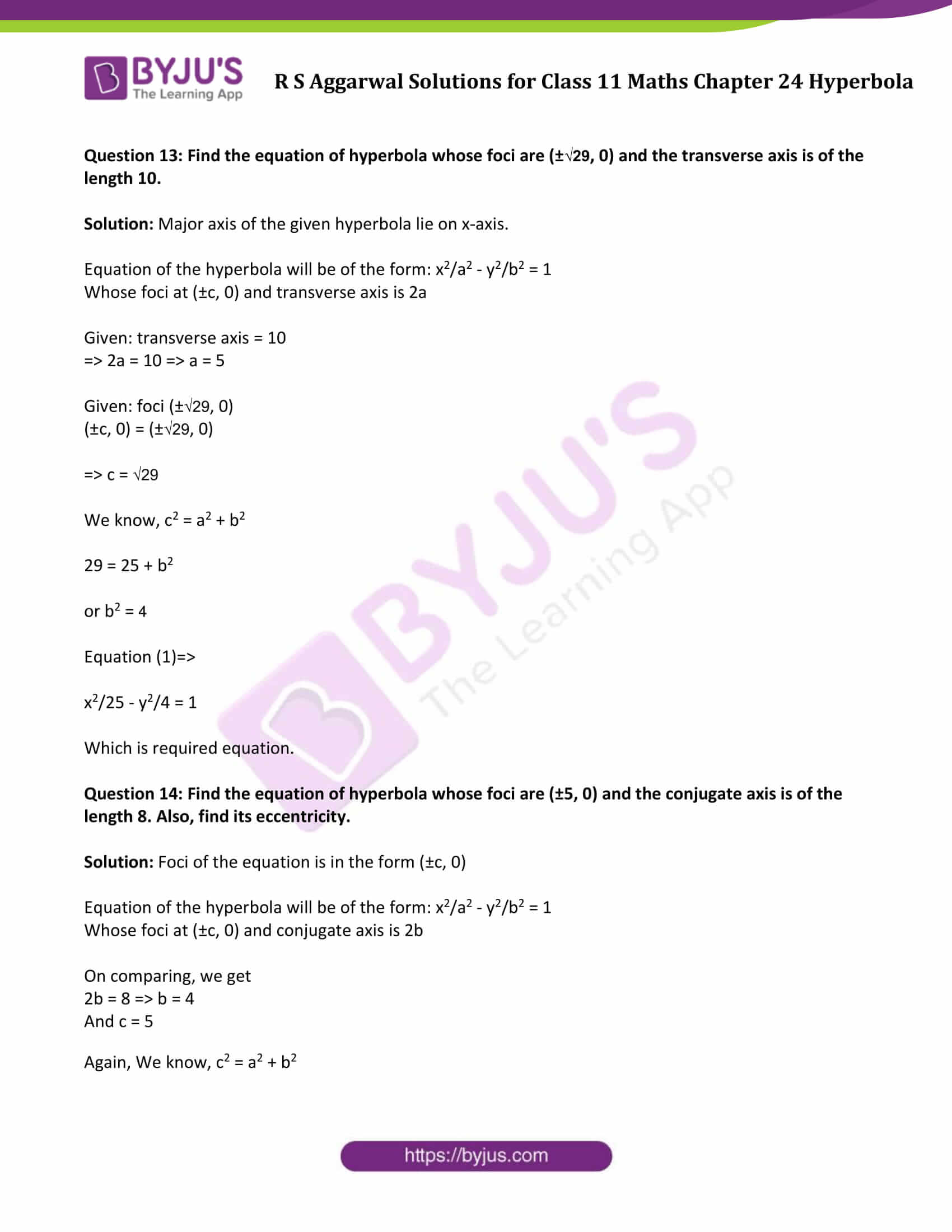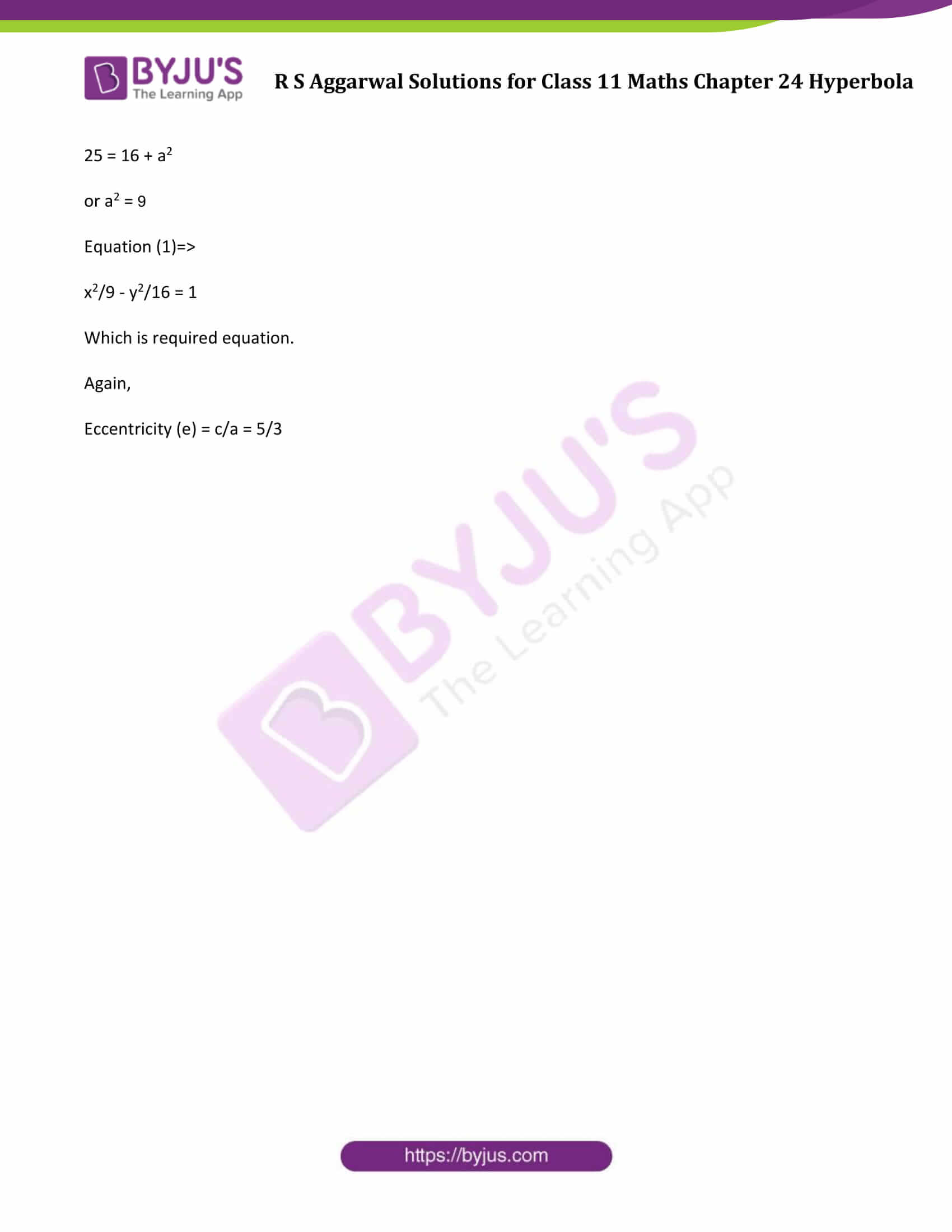### Access Answers to R S Aggarwal Solutions for Class 11 Maths Chapter 24 Hyperbola

Exercise 24 Page No: 771

Question 1: Find the (i) lengths of axes, (ii) coordinates of the vertices, (iii) coordinates of the foci, (iv) eccentricity, and (v) length of the latus rectum of each of the following hyperbola. (Question 1 to question 10)

Horizontal Hyperbola:

For general form of Hyperbola:

x2/a2 – y2/b2 = 1 ……..(1)

 Length of Transverse axis Length of Conjugate axis vertices foci eccentricity latus rectum 2a 2b (±a, 0) (±c, 0) e = c/a 2b2/a

Vertical Hyperbola:

For general form of Hyperbola:

y2/a2 – x2/b2 = 1 ……..(2)

 Length of Transverse axis Length of Conjugate axis vertices foci eccentricity latus rectum 2a 2b (0, ±a) (0, ±c) e = c/a 2b2/a

Question 1: x2/9 – y2/16 = 1

Solution:

Given equation is of the form x2/a2 – y2/b2 = 1

On comparing given equation with (1), we get

a = 3 and b = 4

Then,

c2 = a2 + b2

c2 = 9 + 16 = 25

or c = 5

Now,

(i) Lengths of the axes:

Length of Transverse axis = 2a = 6 units

Length of Conjugate axis = 2b = 8 units

(ii) Coordinates of the vertices: (±a, 0) = (±3, 0)

(iii) Coordinates of the foci: (±c, 0) = (±5, 0)

(iv) Eccentricity: e = c/a = 5/3

(v) Length of the latus rectum: 2b2/a = 2(16)/3 = 32/3 units

Question 2: x2/25 – y2/4 = 1

Solution:

Given equation is of the form x2/a2 – y2/b2 = 1

On comparing given equation with (1), we get

a = 5 and b = 2

Then,

c2 = a2 + b2

c2 = 25 + 4 = 29

or c = √29

Now,

(i) Lengths of the axes:

Length of Transverse axis = 2a = 10 units

Length of Conjugate axis = 2b = 4 units

(ii) Coordinates of the vertices: (±a, 0) = (±5, 0)

(iii) Coordinates of the foci: (±c, 0) = (±√29, 0)

(iv) Eccentricity: e = c/a = √29/5

(v) Length of the latus rectum: 2b2/a = 8/5 units

Question 3: x2 – y2 = 1

Solution:

Given equation is of the form x2/a2 – y2/b2 = 1

On comparing given equation with (1), we get

a = 1 and b = 1

Then,

c2 = a2 + b2

c2 = 1 + 1 = 2

or c = √2

Now,

(i) Lengths of the axes:

Length of Transverse axis = 2a = 2 units

Length of Conjugate axis = 2b = 2 units

(ii) Coordinates of the vertices: (±a, 0) = (±1, 0)

(iii) Coordinates of the foci: (±c, 0) = (±√2, 0)

(iv) Eccentricity: e = c/a = √2

(v) Length of the latus rectum: 2b2/a = 2 units

Question 4: 3x2 – 2y2 = 6

Solution:

Divide each side by 6

x2/2 – y2/3 = 1

Given equation is of the form x2/a2 – y2/b2 = 1

On comparing given equation with (1), we get

a = √2 and b = √3

Then,

c2 = a2 + b2

c2 = 2 + 3 = 5

or c = √5

Now,

(i) Lengths of the axes:

Length of Transverse axis = 2a = 2√2 units

Length of Conjugate axis = 2b = 2√3 units

(ii) Coordinates of the vertices: (±a, 0) = (±√2, 0)

(iii) Coordinates of the foci: (±c, 0) = (±√5, 0)

(iv) Eccentricity: e = c/a = √(5/2)

(v) Length of the latus rectum: 2b2/a = 3√2 units

Question 5: 25x2 – 9y2 = 225

Solution:

Divide both sides by 225

x2/9 – y2/25 = 1

Given equation is of the form x2/a2 – y2/b2 = 1

On comparing given equation with (1), we get

a = 3 and b = 5

Then,

c2 = a2 + b2

c2 = 9 + 25 = 34

or c = √34

Now,

(i) Lengths of the axes:

Length of Transverse axis = 2a = 6 units

Length of Conjugate axis = 2b = 10 units

(ii) Coordinates of the vertices: (±a, 0) = (±3, 0)

(iii) Coordinates of the foci: (±c, 0) = (±√34, 0)

(iv) Eccentricity: e = c/a = √34/3

(v) Length of the latus rectum: 2b2/a = 50/3 units

Question 6: 24x2 – 25y2 = 600

Solution:

Divide both sides by 600

x2/25 – y2/24 = 1

Given equation is of the form x2/a2 – y2/b2 = 1

On comparing given equation with (1), we get

a = 5 and b = 2√6

Then,

c2 = a2 + b2

c2 = 25 + 24 = 49

or c = 7

Now,

(i) Lengths of the axes:

Length of Transverse axis = 2a = 10 units

Length of Conjugate axis = 2b = 4√6 units

(ii) Coordinates of the vertices: (±a, 0) = (±5, 0)

(iii) Coordinates of the foci: (±c, 0) = (±7, 0)

(iv) Eccentricity: e = c/a = 7/5

(v) Length of the latus rectum: 2b2/a = 48/5 units

Question 7: y2/16 – x2/49 = 1

Solution:

Given equation is of the form y2/a2 – x2/b2 = 1

On comparing given equation with (2), we get

a = 4 and b = 7

Then,

c2 = a2 + b2

c2 = 16 + 49 = 65

or c = √65

Now,

(i) Lengths of the axes:

Length of Transverse axis = 2a = 8 units

Length of Conjugate axis = 2b = 14 units

(ii) Coordinates of the vertices: (0, ±a) = (0, ±4)

(iii) Coordinates of the foci: (0, ±c) = (0, ±√65)

(iv) Eccentricity: e = c/a = √65/4

(v) Length of the latus rectum: 2b2/a = 49/2 units

Question 8: y2/9 – x2/27 = 1

Solution:

Given equation is of the form y2/a2 – x2/b2 = 1

On comparing given equation with (2), we get

a = 3 and b = √27 = 3√3

Then,

c2 = a2 + b2

c2 = 9 + 27 = 36

or c = 6

Now,

(i) Lengths of the axes:

Length of Transverse axis = 2a = 6 units

Length of Conjugate axis = 2b = 6√3 units

(ii) Coordinates of the vertices: (0, ±a) = (0, ±3)

(iii) Coordinates of the foci: (0, ±c) = (0, ±6)

(iv) Eccentricity: e = c/a = 2

(v) Length of the latus rectum: 2b2/a = 18 units

Question 9: 3y2 – x2 = 108

Solution:

Divide each side by 108

y2/36 – x2/108 = 1

Which is of the form y2/a2 – x2/b2 = 1

On comparing given equation with (2), we get

a = 6 and b = 6√3

Then,

c2 = a2 + b2

c2 = 36 + 108 = 144

or c = 12

Now,

(i) Lengths of the axes:

Length of Transverse axis = 2a = 12 units

Length of Conjugate axis = 2b = 12√3 units

(ii) Coordinates of the vertices: (0, ±a) = (0, ±6)

(iii) Coordinates of the foci: (0, ±c) = (0, ±12)

(iv) Eccentricity: e = c/a = 2

(v) Length of the latus rectum: 2b2/a = 36 units

Question 10: 5y2 – 9x2 = 36

Solution:

Divide each side by 36, we get

y2/(36/5) – x2/4 = 1

Which is of the form y2/a2 – x2/b2 = 1

On comparing given equation with (2), we get

a = 6/√5 and b = 2

Then,

c2 = a2 + b2

c2 = 36/5 + 4 = 56/5

or c = 2√(14/5)

Now,

(i) Lengths of the axes:

Length of Transverse axis = 2a = 12/√5 units

Length of Conjugate axis = 2b = 4 units

(ii) Coordinates of the vertices: (0, ±a) = (0, ±6/√5)

(iii) Coordinates of the foci: (0, ±c) = (0, ±2√(14/5))

(iv) Eccentricity: e = c/a = √14/3

(v) Length of the latus rectum: 2b2/a = 4√5/3 units

Question 11: Find the equation of hyperbola whose vertices at (±6, 0) and foci at (±8, 0).

Solution:

Vertices are of the form (±a, 0), hence it is a horizontal hyperbola.

So, the equation is of the form: x2/a2 – y2/b2 = 1 …..(1)

Given: vertices of hyperbola (±6, 0)

Here a = 6

Again,

Foci is of the form (±c, 0)

Given: foci of hyperbola at (±8, 0)

⇒ c = 8

Find b:

We know, c2 = a2 + b2

64 = 36 + b2

or b2 = 64 – 36 = 28

Equation (1)⇒

x2/36 – y2/28 = 1

Which is required equation.

Question 12: Find the equation of the hyperbola with vertices at (0, ±5) and foci at (0, ±8).

Solution:

Vertices are of the form (0, ±a), hence it is a vertical hyperbola.

So, the equation is of the form: y2/a2 – x2/b2 = 1 …..(1)

Given: vertices of hyperbola (0, ±5)

Here a = 5

Again,

Foci is of the form (0, ±c)

Given: foci of hyperbola at (0, ±8)

⇒ c = 8

Find b:

We know, c2 = a2 + b2

64 = 25 + b2

or b2 = 39

Equation (1)⇒

y2/25 – x2/39 = 1

Which is required equation.

Question 13: Find the equation of hyperbola whose foci are (±√29, 0) and the transverse axis is of the length 10.

Solution: Major axis of the given hyperbola lie on x-axis.

Equation of the hyperbola will be of the form: x2/a2 – y2/b2 = 1

Whose foci at (±c, 0) and transverse axis is 2a

Given: transverse axis = 10

⇒ 2a = 10 ⇒ a = 5

Given: foci (±√29, 0)

(±c, 0) = (±√29, 0)

⇒ c = √29

We know, c2 = a2 + b2

29 = 25 + b2

or b2 = 4

Equation (1)⇒

x2/25 – y2/4 = 1

Which is required equation.

Question 14: Find the equation of hyperbola whose foci are (±5, 0) and the conjugate axis is of the length 8. Also, find its eccentricity.

Solution: Foci of the equation is in the form (±c, 0)

Equation of the hyperbola will be of the form: x2/a2 – y2/b2 = 1

Whose foci at (±c, 0) and conjugate axis is 2b

On comparing, we get

2b = 8 ⇒ b = 4

And c = 5

Again, We know, c2 = a2 + b2

25 = 16 + a2

or a2 = 9

Equation (1)⇒

x2/9 – y2/16 = 1

Which is required equation.

Again,

Eccentricity (e) = c/a = 5/3

## R S Aggarwal Solutions for Chapter 24 Hyperbola

R S Aggarwal Chapter 24 – Hyperbola, contains the answers to all the questions listed in this chapter. Let us have a look at some of the topics that are being covered here:

• Hyperbola Introduction
• Standard equation of a Hyperbola
• Latus Rectum of a Hyperbola
• Horizontal Hyperbola
• Vertical Hyperbola